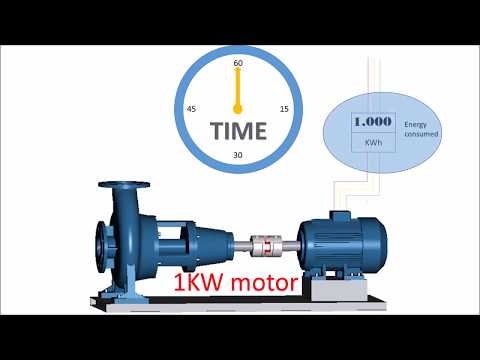### Key difference

The difference between energy and power is that energy is the ability of a body to do work while power is the rate at which specific work is done.

### Energy vs. power

People often consider power and energy to be the same as synonyms, but it is not true. There is a fine line of difference exists between them. Energy is defined as the ability or potential of a body to perform work while power is the rate at which a specific task is performed. This is the crucial difference between them. Further differences will be discussed in detail in this article.

### Comparison Chart

 Energy Power The ability of a body to do work is called energy. The rate of doing work is known as power. Denoted By W P Representation It represents the amount of work done. It represents the speed of work done. SI Unit Joules, i.e. watt/hour Watt, i.e. joule per second Types Energy has various kinds, i.e. thermal, kinetic, potential, light, sound, elastic and electromagnetic etc. Power is divided into human, electric and optical power etc. Storage Energy can be stored. Power cannot be stored. Conversion Energy is a convertible form. Power cannot be converted from one form to another. Equation W= power × time P= energy / time Quantity It is a time quantity. It is an instantaneous quantity.

### What is Energy?

Energy is the capacity or potential of a body to perform some physical work or activity like singing, running, jumping, dancing etc. According to law of conservation of energy, it is stated that energy can neither be formed nor be ruined but it can only be changed from one form to another. An object which is doing work will lose its energy while the one on which work is being done will gain it. Heat energy, light energy, kinetic energy, potential energy, mechanical energy, sound energy, and nuclear energy etc. are different forms of energy. Energy is a time quantity and can also be stored for future use. There are two types of energy sources. One is renewable sources that can be replenished and other is non-renewable sources that cannot be replenished. Several instruments are used to measure energy, e.g. calorimeter (measures heat absorbed or released during any reaction), bolometer (measures the intensity of radiation) and thermometer (measures temperature) etc. It has various units like calories, ergs etc. but the SI unit of energy is joules, i.e. Watt per hour.

### What is Power?

The energy or work gained or spent per unit time is known as power. It is actually the capacity of an object to consume energy, i.e. an object which is using more energy per unit time is more potent than the one which is consuming less. Power cannot be changed from one form to another. It is an instantaneous quantity and cannot be stored. It has different kinds like human power, optical power and electric power etc. The SI unit of power is watt, i.e. joule per second. It measures the rate of transfer of energy.

#### Example

A car A which is travelling 12km in 10 minutes is more powerful as compared to a car B which is moving at a speed of 8km in 10 minutes.

### Key Differences

1. Energy is the capacity of an object to do work while power is the rate at which work is performed.
2. Energy is a time component on the other hand power is an instantaneous quantity.
3. Energy can be stored for future use while power cannot be stored.
4. Energy can change its form, but power cannot be transformed from one way to another.
5. Energy is denoted by “W” while “P” is used as a symbol of
6. SI unit of energy is “Joule” while that of power is “Watt”.

### Comparison Video### Conclusion

According to the above explanations, energy is the transmission of one form to another form while power is the rate of transfer of such forms.